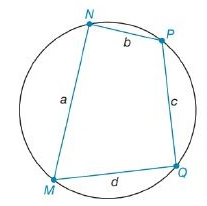Chapter 8.2, Problem 56EElementary Geometry For College St...

7th Edition
Alexander + 2 others
ISBN: 9781337614085

Solutions

Chapter
SectionElementary Geometry For College St...

7th Edition
Alexander + 2 others
ISBN: 9781337614085
Textbook Problem

For the cyclic quadrilateral MNPQ, the sides have lengths a, b, c, and d. If a 2 + b 2 =   c 2 + d 2 , explain why the area of the quadrilateral is A =   a b + c d 2 .To determine

To Show:

The area of the quadrilateral MNPQ is A= ab+cd2 if it is a cyclic quadrilateral with sides a, b, c and d which satisfies the condition a2+b2= c2+d2.

Explanation

Formula Used:

1. Pythagorean theorem for the right angle triangle ABC for the hypotenuse AC,

AC2=AB2+BC2.

2. Area of right triangle =12bh, where b is the base and h is the height of the triangle.

It is given that the cyclic quadrilateral MNPQ with sides a, b, c and d which satisfies the condition a2+b2= c2+d2.

Let’s draw a diagonal between M and P, and MP = k.

Here we can split the quadrilateral MNPQ into two triangles MNP and MQP.

Since the quadrilateral MNPQ is cyclic and satisfies the condition a2+b2= c2+d2, its diagonal MP must be a diameter of the circle.

As MP is a diameter the triangles MNP and MQP are right triangles

Still sussing out bartleby?

Check out a sample textbook solution.

See a sample solution

The Solution to Your Study Problems

Bartleby provides explanations to thousands of textbook problems written by our experts, many with advanced degrees!

Get Started

In Exercises 7-10, solve the equation. 3x210x+8=0

Calculus: An Applied Approach (MindTap Course List)

Convert the following percents to decimals. 334

Contemporary Mathematics for Business & Consumers

True or False: converges.

Study Guide for Stewart's Single Variable Calculus: Early Transcendentals, 8th